()aave## ()aave

بازدید : 700 چهارشنبه 10 دي 1393

This function takes an areal average over an X-Y region. The syntax is:

`aave(expr, xdim1, xdim2, ydim1, ydim2)`

where:

`expr    `- any valid grads expression
``` xdim1   - starting X dimension expression  ``` ``` xdim2   - ending X dimension expression  ``` ``` ydim1   - starting Y dimension expression  ``` ``` ydim2   - ending Y dimension expression  ```

For global averaging, a shorthand may be used:

`aave(expr, global)` or
``` aave(expr, g) ```
is the same as
`aave(expr, lon=0, lon=360, lat=-90, lat=90)`

### Usage Notes

1. In the absence of missing data values, `aave` gives the same result as nested `ave` functions in the X and Y dimensions. The expression

`ave(ave(expr,x=1,x=72),y=1,y=46)`

will produce the same numerical result as

`aave(expr,x=1,x=72,y=1,y=46)`

but the `aave` function is faster more efficient.

2. When there are missing data values, the `aave` function does not return the same result as nested `ave` functions. To see this, consider the small grid:
```
6       18      3       5

10      10      10      10

12      U       U       U

```
where U represents the missing data value. If we apply nested `ave` functions, the inner `ave` will provide row averages of 8, 10, and 12. When the outside `ave` is applied, the result will be an average of 10. When `aave` is used, all the values participate equally (in this case, we are assuming no weights applied to the final average), and the result is 84/9 or about 9.33.

3. The `aave` function assumes that the world coordinates are longitude in the X dimension and latitude in the Y dimension, and does weighting in the latitude dimension by the difference between the sines of the latitude at the northern and southern edges of the grid box. For areal averaging without latitude weighting, use the `amean` function.

4. Both the `aave` and `amean` functions use appropriate weighting to account for unevenly spaced grids.

5. The `aave` function always does its average to the exact boundaries specified, in world coordinates. This is somewhat different from the `ave` function, where the `-b` flag is used to get this behavior. If the boundaries specified via the dimension expressions do not fall on grid boundaries, then the boundary values are weighted appropriately in the average.

6. If grid coordinates are used in the dimensions expressions, then they are converted to world coordinates to determine the exact boundary values. This conversion is done using the scaling of the default file. Note that the conversion is done using the outside grid box boundary, rather than the grid box center. For example:

`asum(expr,x=1,x=72,y=1,y=46)`

Here the boundary would be determined by using the X grid values ranging from 0.5 to 72.5 and Y grid values ranging from 0.5 to 46.5. These four grid boundary values would be converted to world coordinates using the scaling information from the default file. If we assume that `x=1` is 0 degrees longitude and `x=72` is 355 degrees longitude, then the averaging boundary would be -2.5 to 357.5 degrees, which would cover the earth. In the Y dimension, when the boundary is beyond the pole, the `asum` function recognizes this and weights appropriately.

### Examples

1. See the `tloop` function for an example of creating a time series of area averages.

2. An example of taking an area average of data only over land, given a mask grid:

`aave(maskout(p,mask.3(t=1)),x=1,x=72,y=1,y=46) `

In this case, it is assumed the mask grid has negative values at ocean points.

برچسب ها aave , ave , avreag , grads , corolation ,
مطالب مرتبط
ارسال نظر برای این مطلب

نام
ایمیل (منتشر نمی‌شود) (لازم)
وبسایت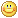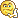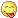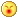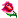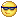نظر خصوصی
مشخصات شما ذخیره شود ؟ [حذف مشخصات] [شکلک ها]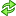کد امنیتی
اطلاعات کاربری
 نام کاربری : رمز عبور :
• فراموشی رمز عبور؟
• لینک دوستان
آرشیو
آمار سایت
• کل مطالب : 155
• کل نظرات : 0
• افراد آنلاین : 1
• تعداد اعضا : 4
• آی پی امروز : 11
• آی پی دیروز : 14
• بازدید امروز : 73
• باردید دیروز : 66
• گوگل امروز : 0
• گوگل دیروز : 0
• بازدید هفته : 322
• بازدید ماه : 2,508
• بازدید سال : 34,346
• بازدید کلی : 155,398
• کدهای اختصاصی